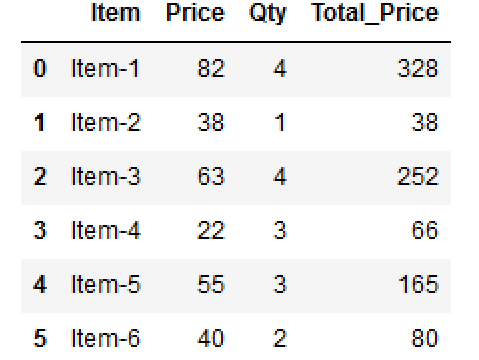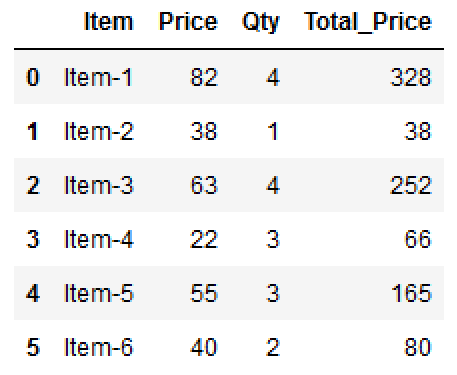Data Science

# New Pandas column based on other columns

One of the most empowering and common operations entails creating a new column in a DataFrame based on the values of existing columns. This invaluable technique opens a gateway to unleashing deeper insights from data, enabling data analysts and scientists to engineer and derive novel features or metrics that complement the original dataset. You can use the following methods to create new column based on values from other columns:

df['Total_Price'] = df.Price * df.Qty
df['Total_Price'] = df.apply(lambda row: row.Price * row.Qty, axis = 1)
df['Total_Price'] = np.multiply(df['Price'], df['Qty'])
df['Total_Price'] = np.vectorize(fx)(df['Price'], df['Qty'])

Lets create a DataFrame..

import pandas as pd import numpy as np df = pd.DataFrame() df['Item'] = ['Item-1', 'Item-2', 'Item-3','Item-4','Item-5','Item-6'] df['Price'] = [82, 38, 63,22,55,40] df['Qty'] = [4, 1, 4,3,3,2] df
Item Price Qty 0 Item-1 82 4 1 Item-2 38 1 2 Item-3 63 4 3 Item-4 22 3 4 Item-5 55 3 5 Item-6 40 2

## Using simple DataFrame multiplication

df['Total_Price'] = df.Price * df.Qty dfItem Price Qty Total_Price 0 Item-1 82 4 328 1 Item-2 38 1 38 2 Item-3 63 4 252 3 Item-4 22 3 66 4 Item-5 55 3 165 5 Item-6 40 2 80

## Using df.apply()

The df.apply() is a powerful and versatile method used to apply a function along either the rows or columns of a DataFrame. This method allows data professionals to perform custom operations or calculations on the DataFrame's data, facilitating data transformation, cleaning, or feature engineering with efficiency and precision.

The syntax for df.apply() is as follows:

df.apply(func, axis=0)
df['Total_Price'] = df.apply(lambda row: row.Price * row.Qty, axis = 1) df

Example:

Item Price Qty Total_Price 0 Item-1 82 4 328 1 Item-2 38 1 38 2 Item-3 63 4 252 3 Item-4 22 3 66 4 Item-5 55 3 165 5 Item-6 40 2 80

## Using np.multiply()

The np.multiply() is a fundamental function used to perform element-wise multiplication on arrays. This versatile function allows data professionals to multiply corresponding elements of one or more arrays, aligning their dimensions for consistent and accurate calculations.

The syntax for np.multiply() is as follows:

np.multiply(x1, x2, ...)

Example:

df['Total_Price'] = np.multiply(df['Price'], df['Qty']) df
Item Price Qty Total_Price 0 Item-1 82 4 328 1 Item-2 38 1 38 2 Item-3 63 4 252 3 Item-4 22 3 66 4 Item-5 55 3 165 5 Item-6 40 2 80

## Using vectorize arbitrary function

You can vectorize an arbitrary function using the np.vectorize() function. This process involves transforming a Python function that operates on scalars into a vectorized function that can work element-wise on arrays. By vectorizing a function, you can apply it to NumPy arrays directly, allowing for efficient element-wise computations without the need for explicit loops.

The np.vectorize() function takes an input function and returns a new function that can operate on arrays. It uses NumPy's broadcasting capabilities to apply the function to each element of the input arrays, making it well-suited for element-wise operations.

Example:

def fx(x, y): return x*y df['Total_Price'] = np.vectorize(fx)(df['Price'], df['Qty']) dfItem Price Qty Total_Price 0 Item-1 82 4 328 1 Item-2 38 1 38 2 Item-3 63 4 252 3 Item-4 22 3 66 4 Item-5 55 3 165 5 Item-6 40 2 80

### Conclusion

The process of crafting a new Pandas column based on other columns constitutes an artful fusion of creativity and data science acumen. By judiciously using the vast array of Pandas' data transformation capabilities, data professionals can transcend the boundaries of raw data and forge a tapestry of insightful metrics and features that unravel the true potential of the dataset. This approach stands as an indispensable instrument in the data analyst's toolkit, exemplifying the boundless potential of Pandas in transforming data into actionable knowledge and driving data-driven solutions with ingenuity and finesse.